# 算法分析：方阵的主对角线之上称为“上三角”。

1 2 3
6 4
5

1 2 3 4
9 10 5
8 6
7

1 2 3 4 5
12 13 14 6
11 15 7
10 8
9

## 思路解析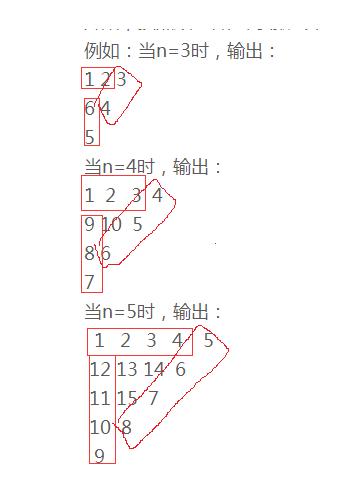1 2 3 4 5 6
15 16 17 18 7
14 21 19 8
13 20 9
12 10
11
1 2 3 4 5 6 7 8 9 10 11 12 13 14 15 为最外层第一层，16 17 18 19 20 21为第二层

        //n为我们人为输入的数
//定义一个二维数组存放变量值
int a[][] = new int[n][n];
for (int i = 0; i < n; i++) {
for (int j = i; j < n - 1 - i * 2; j++)

{
a[i][j] = k;
k++;
}
for (int j = i; j < n - i * 2 - 1; j++) {
a[j][n - 1 - i - j] = k;
k++;
}
for (int j = i; j < n - 2 * i - 1; j++) {
a[n - 1 - i - j][i] = k;
k++;
}
}


a =null a =null a=null a=null
a =null a =null a=null a=null
a =null a =null a=null a=null
a =null a =null a=null a=null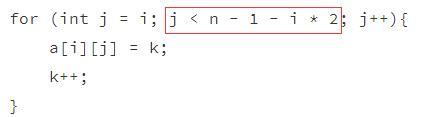a =001 a =002 a=003 a=000
a =000 a =000 a=000 a=000
a =000 a =000 a=000 a=000
a =000 a =000 a=000 a=000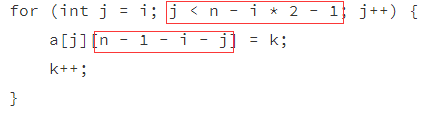a =001 a =002 a=003 a=004
a =000 a =000 a=005 a=000
a =000 a =006 a=000 a=000
a =000 a =000 a=000 a=000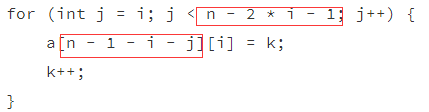a =001 a =002 a=003 a=004
a =009 a =000 a=005 a=000
a =008 a =006 a=000 a=000
a =007 a =000 a=000 a=000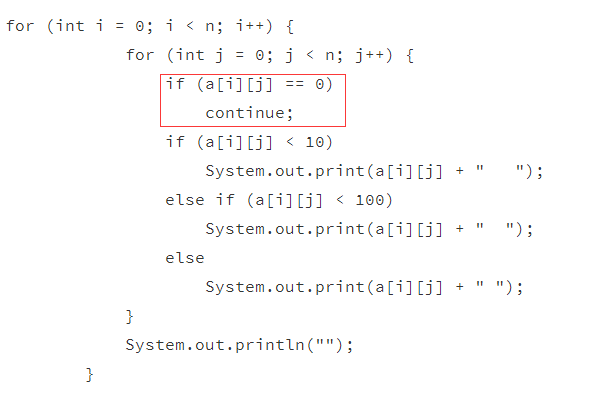“

a =001 a =002 a=003 a=004
a =009 a =000 a=005
a =008 a =006
a =007

 if ((n - 1) % 3 == 0)
a[(n - 1) / 3][(n - 1) / 3] = k;

2句代码解决这个问题。

## 最终完整代码

public class Test{
public static void main(String[] args) {

//键盘输入
Scanner sc = new Scanner(System.in);
System.out.println("put n!");
//n代表当前的循环数
int n = sc.nextInt();
int k = 1;
//定义一个二维数组存放变量值
int a[][] = new int[n][n];
for (int i = 0; i < n; i++) {
for (int j = i; j < n - 1 - i * 2; j++)

{
a[i][j] = k;
k++;
}
for (int j = i; j < n - i * 2 - 1; j++) {
a[j][n - 1 - i - j] = k;
k++;
}
for (int j = i; j < n - 2 * i - 1; j++) {
a[n - 1 - i - j][i] = k;
k++;
}
}
if ((n - 1) % 3 == 0)
a[(n - 1) / 3][(n - 1) / 3] = k;
for (int i = 0; i < n; i++) {
for (int j = 0; j < n; j++) {
if (a[i][j] == 0)
continue;
if (a[i][j] < 10)
System.out.print(a[i][j] + "   ");
else if (a[i][j] < 100)
System.out.print(a[i][j] + "  ");
else
System.out.print(a[i][j] + " ");
}
System.out.println("");
}
}
}`